Next: Histograms Up: Output Previous: Number and Energy Spectra

# Energy Density

The function energy() calculates the components of the energy density. The energy density is calculated for all fields regardless of the value of noutput_flds. The output is in two files, one for the components of energy density and one for monitoring energy conservation.

The components are output to a file called energy_ext. The number of columns in this file varies with the number of fields and with the number of terms in the potential. Specifically, the first column contains the time, the next nflds columns contain the kinetic energy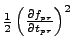, the next nflds columns contain the gradient energy, and the remaining columns contain the potential terms. The kinetic and gradient energies are calculated by energy(), whereas the potential energy is calculated by a model-specific function called potential_energy() in model.h. The output is in program units, meaning(5.41)

where(5.42)

is the physical energy density and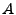,,, and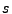are the rescaling parameters defined in equation (6.2). Angle brackets denote averages over the grid. Plugging in the rescalings gives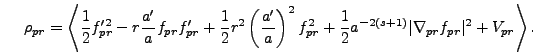(5.43)

The second file generated by energy() is called conservation_ext. The first column contains the time and the second column contains a quantity used to monitor energy conservation. For the case of no expansion (Minkowski space) this quantity is simply the ratio of total energy density to energy density at the beginning of the run. Of course this quantity should remain close to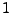throughout the run. In an expanding universe the situation is a little more complicated because energy density is not conserved, but rather decreases in a way determined by the expansion rate and the equation of state of the fields. This redshifting of energy is described by the continuity equation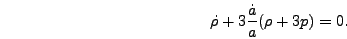(5.44)

Equation (5.44) can be derived from the Friedmann equations, so if these are being solved exactly then the continuity equation will be obeyed as well. So a simple way to check energy conservation in an expanding universe is to use one of the Friedmann equations, equations (6.19) and (6.20) to evolve the scale factor and then check if the other is being satisfied as well. In practice the program uses a combination of these two equations so either one can be used as a check of energy conservation. We use equation (6.20), i.e.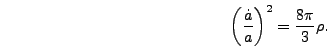(5.45)

So for an expanding universe the second column of the conservation file contains the ratio of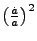to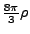. To express this in terms of program variables we use equations (5.2) and (5.41) to give(5.46)

As in the case of no expansion this quantity should remain close tothroughout the run. We have generally found that the deviation of this ratio from one is comparable to the lack of energy conservation for runs done with the same models without expansion. We have also checked that using the second Friedmann equation, equation (6.19), gives essentially the same results as this method.

In the case of fixed power-law expansion the conservation file is not created.Next: Histograms Up: Output Previous: Number and Energy Spectra